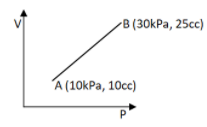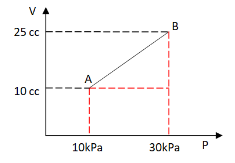Courses
Courses for Kids
Free study material
Offline Centres
MoreLast updated date: 27th Nov 2023
Total views: 277.5k
Views today: 3.77k

# Find the work done for the process shown in the figure(a) 1J(b) 1.5J(c) 4.5J(d) 0.3JVerified
277.5k+ views
Hint: The work done of the process is found by calculating the area under the curve. Redraw the diagram and extend lines to the X and Y axis from points A and B. Calculate the total area by dividing it into smaller areas. In this figure divide it into a triangular and rectangular area and then sum the values of the area. The total area will give the work done.

Formula used: $Area{\text{ }}of{\text{ }}rectangle = {\text{ }}length{\text{ }}x{\text{ }}breadth$ ; $Area{\text{ }}of{\text{ }}triangle{\text{ }} = \;\dfrac{1}{2}*{\text{ }}Base{\text{ * }}height$

Complete step-by-step solution:$P - {\text{ }}Pressure$
$Pa - {\text{ }}Pascal{\text{ }}(Unit{\text{ }}of{\text{ }}pressure)$
$V - {\text{ }}Volume$
$cc - {\text{ }}cubic{\text{ }}centimeter{\text{ }}\left( {Unit{\text{ }}of{\text{ }}volume} \right)$
The work done is calculated by summing the area under the line AB. So from the diagram, the sum of the area (area 0f the rectangle + area of the triangle) will give the work done for the process.
Given: Initial volume =$10{\text{ }}cc$ ; Final volume = $25{\text{ }}cc$
Initial Pressure =$10kPa$ ; Final pressure = $30kPa$
To find: work done
Area of the rectangle (from the diagram) = Length x breadth
Substituting the values, = $\left( {30 - 10} \right){\text{* }}10$
$= {\text{ }}20{\text{ * }}10$
$Area{\text{ }}of{\text{ }}rectangle\;\;\; = {\text{ }}200kPa.cc$
Area of the triangle (from the diagram) = $\;\dfrac{1}{2}{\text{* }}Base{\text{ * }}height$
$= \;\dfrac{1}{2}*{\text{ }}10{\text{ * }}20$
Simplifying, Area of triangle= $100$ kPa.cc
The work done is the sum of the area,
Therefore, Work done= Area of rectangle + Area of triangle
Substituting the values, = $200$ kPa.cc + $100$ kPa.cc
Adding,= $300$ kPa.cc
To convert cubic centimeter to cubic meter, multiply by ${10^{ - 6}}$ (1 cubic centimeter= ${10^{ - 6}}$ cubic meter)
Therefore, Work done = $300{\text{ * }}{10^{ - 6}}$ kPa.m3
Since $kPa = {\text{ }}1000$ Pa and multiplying, Work done= $0.3$ Pa.m3
Since$Pa.{m^3} = {\text{ }}J$ , Work done=$0.3$J (J- Joules)
Hence option (D) W= $0.3$J is the right answer.

Note: The energy that is utilized to move an object against force is called work. The work done by a gas depends on both the initial and final state of a gas. For a pressure-volume graph, the area under the curve gives the work done by the gas.